# Using the PALM4MSA-MHTP Algorithm

In this live script we shall see how to use the PALM4MSA-MHTP algorithm. A live script has already been written on the Hierarchical PALM4MSA algorithm and its wrappers and is a prerequisite to the reading of this live script.
The PALM4MSA-MHTP is a variant of PALM4MSA in which intervenes the Multilinear Hard Tresholdhing Pursuit algorithm (MHTP).
The interest of this variant is to avoid the situation in which PALM4MSA tends to stuck on some matrix supports without any way out. MHTP allows to explore the support more freely and hopefully find a more accurate factorization at the cost of just a few more dozens iterations of the gradient descent algorithm.
For more information on the theory, you can read the following paper in which is treated the particular case of the BHTP (Bilinear HTP, that is running the MHTP on only two factors).
 Quoc-Tung Le, Rémi Gribonval. Structured Support Exploration For Multilayer Sparse Matrix Fac- torization. ICASSP 2021 - IEEE International Conference on Acoustics, Speech and Signal Processing, Jun 2021, Toronto, Ontario, Canada. pp.1-5. hal-03132013.

## Configuring and Running PALM4MSA-MHTP

This variant works very similarly to a classic run of PALM4MSA, that is with at least the same set of parameters. The main difference is that periodically (in term of PALM4MSA iterations) the MHTP algorithm is launched to renew each layer of the Faust being refined.
Hence running the PALM4MSA-MHTP needs two sets of parameters: ParamsPalm4MSA and MHTPParams objects. The former should not be really new if you are used to PALM4MSA, the latter is dedicated to the configuartion of the MHTP part of PALM4MSA-MHTP.
The arguments to configure MHTPParams are basically:
• num_its: the number of iterations MHTP runs on each layer of the Faust. Remember that this number of iterations is for each factor. If you have two factors the overall number of iterations is 2 x num_its (exactly as it is for PALM4MSA).
• constant_step_size and step_size: that determines if the MHTP gradient descent will be ran according to a constant step size, and in that case how long is the step size. By default, the step size is not constant and recomputed dynamically with the Lipschitz coefficient as in PALM4MSA. In most case, it is recommended to not use a constant step size to achieve a better loss function.
• palm4msa_period: which governs how many times to evenly run the MHTP algorithm inside PALM4MSA itself. By default, the value is 50. It means that for example if PALM4MSA is running for 200 iterations, MHTP will run 4 times: at iterations 0, 49, 99, 149 and 199 of PALM4MSA. Every time it runs MHTP will run for num_its iterations.
• updating_lambda: this boolean when set to True allows to update the scale factor of the Faust (the same one that is used in PALM4MSA) in the end of each iteration of MHTP.
So let's run PALM4MSA-MHTP on a small example: we propose to factorize a 500x32 matrix into two factors.
First we configure PALM4MSA as usual:
• The number of iterations of PALM4SA with the StoppingCriterion (here 200 iterations).
• Then we define the constraints / projectors to use, here the SPLIN projector for the first factor of size 500x32 into which we want to count 5 nonzeros per row and the NORMCOL projector for the second factor in which each column must be normalized.
import matfaust.factparams.*
import matfaust.proj.*
projs = { splin([500, 32], 5), normcol([32, 32], 1.0) };
stop_crit = StoppingCriterion(200);
param = ParamsPalm4MSA(projs, stop_crit);
Second we define the MHTPParams structure to configure the MHTP pass of PALM4MSA-MHTP
Hence we define the arguments described above: num_its, etc. We let all of them to their default values so there is not much to do.
import matfaust.factparams.MHTPParams
mhtp_param = MHTPParams()
mhtp_param =
MHTPParams with properties: num_its: 50 constant_step_size: 0 step_size: 1.0000e-03 palm4msa_period: 1000 updating_lambda: 1
It's now time to run the PALM4MSA-MHTP algorithm passing the two structures of parameters. Before we generate a random matrix M to factorize.
import matfaust.fact.palm4msa_mhtp
M = rand(500, 32);
F = palm4msa_mhtp(M, param, mhtp_param)
F =
Faust size 500x32, density 0.22025, nnz_sum 3524, 2 factor(s): - FACTOR 0 (real) SPARSE, size 500x32, density 0.15625, nnz 2500 - FACTOR 1 (real) SPARSE, size 32x32, density 1, nnz 1024
As you see it's pretty similar to running PALM4MSA, which we could have done with the following code.
import matfaust.fact.palm4msa
G = palm4msa(M, param)
G =
Faust size 500x32, density 0.22025, nnz_sum 3524, 2 factor(s): - FACTOR 0 (real) SPARSE, size 500x32, density 0.15625, nnz 2500 - FACTOR 1 (real) DENSE, size 32x32, density 1, nnz 1024
We can verify that the results are however not the same:
format long
mhtp_error = norm(full(F)-M)/norm(M)
mhtp_error =
0.093802635735114
palm4msa_error = norm(full(G)-M)/norm(M)
palm4msa_error =
0.093797104961271
They are very close though! In the next part of this live script we'll demonstrate how PALM4MSA-MHTP can really enhance the accuracy of the Faust approximate and will do that on the MEG matrix (this matrix is also discussed and factorized in ).

## Factorizing the MEG matrix using the PALM4MSA-MHTP algorithm

The MEG (for magnetoencephalography) matrix is also used in  to compare PALM4MSA and PALM4MSA-MHTP performance.
The goal is to factorize the MEG matrix as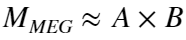with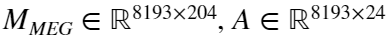and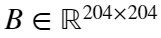. A and B are subject to sparsity constraints. Here we'll test only one sparsity configuration of the two factors (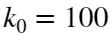and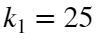being respectively the per-row number of nonzeros of A and B).
Let's load the MEG matrix which is embedded in FAµST data package (which should be downloaded automatically).
MEG = matrix;
size(MEG)
ans = 1×2
8193 204
Going ahead we set the PALM4MSA parameters:
import matfaust.proj.*
import matfaust.factparams.*
k0 = 100;
k1 = 25;
% the relevant projectors for our sparsity constraints
projs = {splin([8193, 204], k0), splin([204, 204], k1)};
% the number of iterations of PALM4MSA
stop_crit = StoppingCriterion(2000);
param = ParamsPalm4MSA(projs, stop_crit);
param.use_csr = false;
It remains the MHTPParams configuration (it's easy, we use the default parameters) :
mhtp_p = MHTPParams();
Now we are able to launch PALM4MSA and PALM4MSA-MHTP and compare the errors: the computation takes some time, it can last about 30 minutes.
import matfaust.fact.*
F_palm4msa = palm4msa(MEG, param);
F_mhtp = palm4msa_mhtp(MEG, param, mhtp_p);
err_palm4msa = norm(F_palm4msa-MEG) / norm(MEG)
err_palm4msa_mhtp = norm(F_mhtp-MEG) / norm(MEG)
As you see the MHTP variant is twice accurate than PALM4MSA on this configuration.

## Using the Hierarchical PALM4MSA-MHTP algorithm

Exactly the same way you can use the hierarchical factorization with PALM4MSA, it is possible to use the function matfaust.fact.hierachical_mhtp to run a hierarchical factorization based on PALM4MSA-MHTP instead of simply PALM4MSA.
The launch of the algorithm function is very similar, you just need to add a MHTPParams instance to the argument list.
Let's show how with this simple example:
import matfaust.fact.*
import matfaust.factparams.*
import matfaust.proj.*
M = rand(500, 32);
fact_projs = {splin([500, 32], 5), sp([32, 32], 96), sp([32, 32], 96)};
res_projs = {normcol([32, 32], 1), sp([32, 32], 666), sp([32, 32], 333)};
stop_crit1 = StoppingCriterion(200);
stop_crit2 = StoppingCriterion(200);
% 50 iterations of MHTP will run every 100 iterations of PALM4MSA
% (each time PALM4MSA is called by the hierarchical algorithm)
mhtp_params = MHTPParams('num_its', 50, 'palm4msa_period', 100);
param = ParamsHierarchical(fact_projs, res_projs, stop_crit1, stop_crit2);
F = hierarchical_mhtp(M, param, mhtp_params)
mex_params = struct with fields:
nfacts: 4 cons: {2×3 cell} niter1: 200 niter2: 200 sc_is_criterion_error: 0 sc_error_treshold: 0.3000 sc_max_num_its: 10000 sc_is_criterion_error2: 0 sc_error_treshold2: 0.3000 sc_max_num_its2: 10000 nrow: 500 ncol: 32 fact_side: 0 update_way: 0 verbose: 0 init_lambda: 1 use_csr: 1 packing_RL: 1 norm2_threshold: 0 norm2_max_iter: 0 step_size: 1.0000e-16 constant_step_size: 0 mhtp_num_its: 50 mhtp_constant_step_size: 0 mhtp_step_size: 1.0000e-03 mhtp_palm4msa_period: 100 mhtp_updating_lambda: 1
Faust::hierarchical: 1 Faust::hierarchical: 2 Faust::hierarchical: 3 - FACTOR 0 (real) SPARSE, size 500x32, density 0.15625, nnz 2500 - FACTOR 1 (real) SPARSE, size 32x32, density 0.09375, nnz 96 - FACTOR 2 (real) SPARSE, size 32x32, density 0.09375, nnz 96 - FACTOR 3 (real) SPARSE, size 32x32, density 0.3125, nnz 320
This live script is ending here. Please note that although th e article  tackles the optimization problem of approximately factorizing a matrix in two sparse factors with the Bilinear Hard Tresholding Pursuit (BHTP) algorithm, the MHTP is a generalization to N factors that needs further experiences to be mature. Hence the function palm4msa_mhtp and moreover the function hierarchical_mhtp should be considered as experimental code and might evolve significantly in the future.
Thanks for reading this live script! Many other are available at faust.inria.fr.
Note: this live script was executed using the following matfaust version:
matfaust.version()